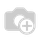# How i can Apply onchange method on one2many field ?

for example i have three model one is master & two is detail models

Model A

Model B

Model C

Model A is master Model B & Model C is detail

i hav filed in Model B type when i change type then i want to apply domain on Model C
how i can do this any helpHi Usman,

Try following Code

class MasterClass(models.Model):
_name = 'master.class'

o2m_f1 = fields.One2Many('o2m.table1', 'field')
o2m_f2 = fields.One2Many('o2m.table2', 'field')

Class O2mTable1(models.Model):
_name = 'o2m.table1'

name = fields.Char()
field = fields.Many2one('master.class')

@api.onchange('name')
def trigger_o2m_change():

Class O2mTable2(models.Model):
_name = 'o2m.table2'

name = fields.Char()
field = fields.Many2one('master.class')

@api.onchange('name')
def trigger_o2m_change():